# Quick Questions - Fractions, Decimals, Percents, & Ratios Notes | Study Practice Questions for GMAT - GMAT

## GMAT: Quick Questions - Fractions, Decimals, Percents, & Ratios Notes | Study Practice Questions for GMAT - GMAT

The document Quick Questions - Fractions, Decimals, Percents, & Ratios Notes | Study Practice Questions for GMAT - GMAT is a part of the GMAT Course Practice Questions for GMAT.
All you need of GMAT at this link: GMAT

Section - 1
Ques 1: Convert 45% to a decimal.
Ans:
0.45: 45% becomes 0.45

Ques 2: Convert 0.20 to a percent.
Ans:
20%: 0.20 becomes 20%

Ques 3: Convert 4 /5 to a percent.
Ans: 80%:
Step 1: 4 -s- 5 = 0.8
Step 2: 0.8 becomes 80%

Ques 4: Convert 13.25% to a decimal.
Ans:
0.1325: 13.25% becomes 0.1325

Ques 5: Convert 6 /2 0 to a percent.
Ans:
30%: Step 1: 6 -s- 20 = 0.30
Step 2: 0.30 becomes 30%

Ques 6: Convert 0.304 to a percent.
Ans:
30.4%: 0.304 becomes 30.4%

Ques 7: Convert 0.02% to a decimal.
Ans:
0.0002: 0.02% becomes 0.0002

Section - 2
Simplify the following expressions. Answers in the explanation may appear in more than one form (e.g.,fraction and decimal).
Ques 8: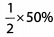Ans: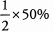Convert percent to fraction because fractions are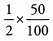easier to multiply than percents.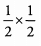Simplify before you multiply.
1/4

Ques 9: .2 5 % -0 .1
Ans: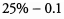Convert decimal to percent because decimals that end inthe tenths or hundredths digit are easy to convert to percents.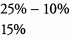Ques 10: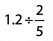Ans
: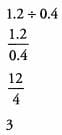Multiply by 10/10 to convert decimals to integers.

The document Quick Questions - Fractions, Decimals, Percents, & Ratios Notes | Study Practice Questions for GMAT - GMAT is a part of the GMAT Course Practice Questions for GMAT.
All you need of GMAT at this link: GMATUse Code STAYHOME200 and get INR 200 additional OFF

## Practice Questions for GMAT

10 docs|48 tests

Track your progress, build streaks, highlight & save important lessons and more!

,

,

,

,

,

,

,

,

,

,

,

,

,

,

,

,

,

,

,

,

,

,

,

,

,

,

,

,

,

,

;